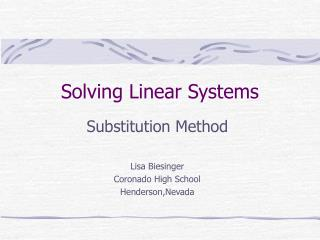Download PresentationSolving Linear Systems

# Solving Linear Systems

Download Presentation## Solving Linear Systems

- - - - - - - - - - - - - - - - - - - - - - - - - - - E N D - - - - - - - - - - - - - - - - - - - - - - - - - - -
##### Presentation Transcript

1. Solving Linear Systems Substitution Method Lisa Biesinger Coronado High School Henderson,Nevada

2. Linear Systems • A linear system consists of two or more linear equations. • The solution(s) to a linear system is the ordered pair(s) (x,y) that satisfy both equations.

3. Example • Linear System: • The solution will be the values for x and y that make both equations true.

4. Solving the System • Step 1: Solve one of the equations for either x or y. • For this system, the first equation is easy to solve for x because the coefficient of x is equal to 1.

5. Organizing Your Work • Set up 2 columns on your paper. • Place one equation in each column. • Write the equation we are using first in column 1, and the other equation in column 2.

6. Now we will solve for x in column 1. Solving by Substitution

7. Subtract 2y from both sides. Solving by Substitution

8. Solving the System • Step 2: Substitute your answer into the other equation in column 2. • Substituting will eliminate one of the variables in the equation.

9. Always use parenthesis when substituting an expression with two terms. Solving by Substitution

10. Solving the System • Step 3: Simplify the equation in column 2 and solve.

11. Use the distributive property and combine like terms. Solving by Substitution

12. Solve for y. Solving by Substitution

13. Solving the System • Step 4: Substitute your answer in column 2 into the equation in column 1 to find the value of the other variable.

14. Substitute for y and solve for x. Solve for x. Solving by Substitution

15. The Solution • The solution to this linear system is and . • The solution can also be written as an ordered pair

16. Almost FinishedChecking Your Solution • Check your answer by substituting for x and y in both equations.

17.  Checking Your Answer

18. Problem #1: Problem#2 Problem #3 Answers: #1 #2 #3 Additional Examples